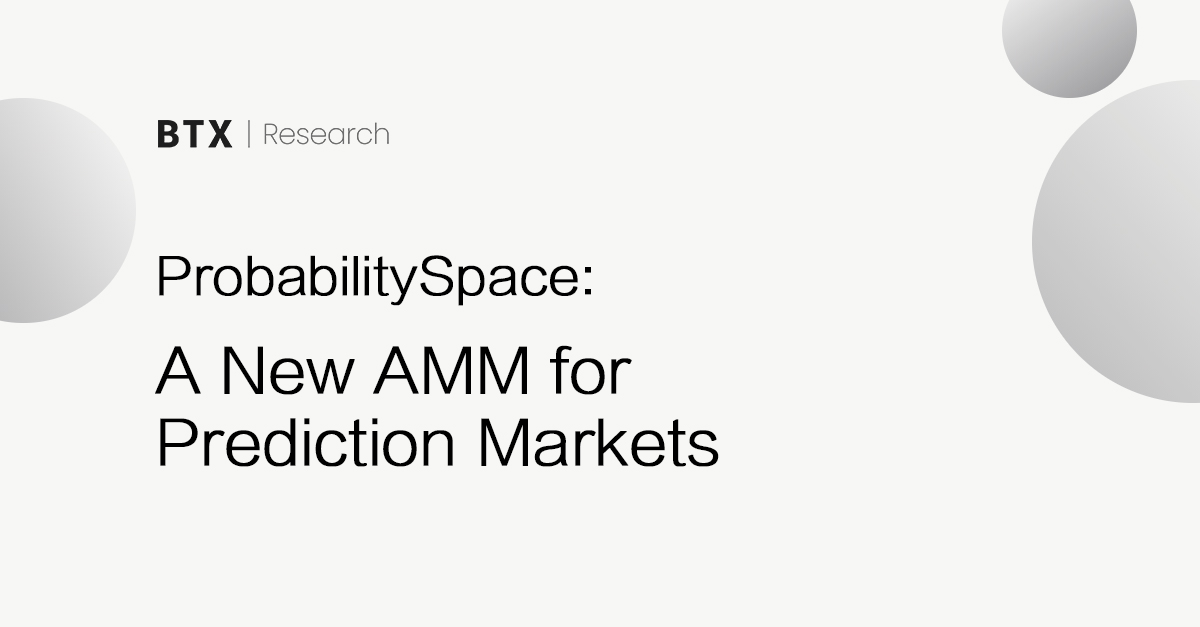## ProbabilitySpace: A New AMM for Prediction Markets

April 20, 2023TL;DR

Traditional Constant Function Market Makers (CFMMs) are not well-suited for applications such as prediction markets. They are time-independent which means no liquidity distribution adjustment and liquidity provider protection, which are needed for prediction markets.

In this paper, we present a time-dependent AMM that is similar in form to YieldSpace, which spreads the liquidity to the “edges” as time gets closer to maturity. This results in minimum amounts of YES and NO shares at any time, which protects liquidity providers.

We call it ProbabilitySpace.

While YieldSpace aims to price a constant interest rate given a ratio of reserves, ProbabilitySpace tries to price probabilities of YES and NO with the underlying volatility and time of a stochastic process.

#### 1. Derivation of the AMM

We want the AMM to spread the liquidity to 100% YES and 100% NO as time gets closer to maturity.

Here we choose the YES and NO probabilities to follow a binary option, as the natural log of the ratio of reserves of YES and NO shares follows a normal distribution.

The probability of YES and NO should increase or decrease as time goes to maturity.

The probability of NO can be represented as:

where N(d) is the CDF of Normal Distribution N(0,1).

We can also rewrite the first equation as:

which means the price, as the ratio of the probability of NO and YES, increasing to infinity or decreasing to zero as time gets closer to maturity.

Using approximation formulas from this paper, we can perform one-parameter and two-parameter approximations, which make the differential equation easier to solve with an analytical solutions.

One-parameter approximation

Approximate N(d) as:

Without loss of generality, let

Then

Thus, at time t

## Two-parameter approximation

Approximate N(d) as:

Without loss of generality, let

Then

The second-order approximation generates a differential equation that is difficult to solve as well. Therefore, for the rest of the article, we use one-parameter approximation.

The AMM we arrive at:

looks very similar to YieldSpace except t is replaced by 1/sqrt(t).

#### 2. Liquidity fingerprint

We can analyze the evolution of the liquidity distribution by using techniques in this article, measuring the liquidity with respect to the price tick.

The marginal price of the AMM is:

y can be expressed as a function of P:

P can be expressed in terms of ticks:

Therefore

As we can see from the Desmos illustration, as time goes to maturity at 0, the AMM spreads the liquidity to 100% YES and 100% NO as intended.

#### 3. Non-constant s

The above calculations assumed s stays constant over time.

s stays constant only if x and y stays at s.

For instance, if x = 2, y = 1:

At t = 0.5, s = ~1.38

At t = 0.25, s = ~1.33

Thus, here we discuss the evolution of the AMM when s is not constant.

Specifically, we define the marginal price of x at t = t0 as P0, the s value at t = t0 as s0.

Using the above two formulas, we can solve for x0 and y0:

Assume when t < t0, x = x0, y = y0.

Then we can express s as a function of t:

Plug in x0 and y0, solve for s:

Substituting s in the following equation:

The AMM is flattened as time goes to maturity, which suggests there is a minimum of YES and NO shares no matter what, for LP protection.

#### 4. xmin and ymin

xmin and ymin refer to the minimum amounts of YES and NO shares the AMM allows at any time.

Assume when t < t0, x = x0, y = y0.

If t ＞= 1, xmin = ymin = 0.

If t < 1,

The maximum amounts of shares that can be swapped out:

At t = 0,

because the AMM goes through (x0,y0).

#### 5. Liquidity provider protection

Liquidity providers are protected due to the designed time evolution of the AMM:

• The marginal price becomes worse as time is closer to maturity.
• There is a minimum amount of YES and NO shares that cannot be swapped out.

Assume when t < t0, x = x0, y = y0.

% protected

If YES,

If NO,

Divergence loss (geometrically)

Suppose there is a trade that happens at time t that brings the marginal price from P to Pk.

Therefore,

Divergence loss (practically)

Suppose there is a trade that happens at time t that brings the marginal price from P to Pk.

This is the first trade since t0 and the last trade till maturity.

If YES,

If NO,

This Desmos illustration graphs the practical divergence loss with respect to t and k. The practical divergence loss decreases as time goes closer to maturity.

#### 6. Balancer weight method

There are alternative AMMs that can be generated mathematically which evolve similarly to the AMM we derived above.

In Balancer,

Therefore we can choose to adjust the weights to satisfy:

One-parameter approximation

If we keep y/x in the exponent constant,

If y/x in the exponent varies,

We assume the AMM goes through (x0,y0) at t = t0, and when t < t0, x = x0, y = y0.

The second function, in which y/x in the exponent varies, surprisingly behaves very similar to:

However, the two functions do not satisfy the relation -dy/dx = (y/x) ^ (1/sqrt (t)). Specifically, the first function only satisfies the relation at (x0, y0).Arbitrum and the Dawn of a New Era: Navigating Ethereum’s Cancun Upgrade and the Future of \$ARBETH ShangHai UpgradeProbabilitySpace: A New AMM for Prediction Markets